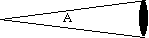Angular Size

Use one eye to look at a large distant object like a car.

Use a finger held out at arms length to point at one side of the object and then move the finger to point at the other side of the object. To move your finger from one side to the other side of the object you have to swing your arm through an angle. This angle is the angle subtended by the object. It is the angular size of the object.

The angle is often measured in degrees.

There are 360 degrees in a complete circle.

The angle can also be measured in radians, there are 2 pi radians in a complete circle.

To find the angular size of an object in radians, A, draw a circle through the object with your eye at the center of the circle. The angle is the length of the arc of the circle obscured by the object, d in distance units such as meters, divided by the radius of the circle, R in the same units.

A = d/R

If the object is small or far away, that is if d/R is small, you can replace the length of the arc of the circle with the distance across the object, since the two will be nearly the same length.A dark ellipse subtends and angle A in radians.

To find the angular size in degrees, a, find the angular size in radians and multiply by 360/2 pi

a = A * 360/2 pi

Another way to find the angular size is to create a triangle with the base of the triangle the distance from your eye to the object and the height of the triangle half of the length of the object and note that

A = 2 arctangent (d/2R)

If the angle A is measured in radians then the arctangent is approximately equal to (d/2R) so the answer is the same as the answer given above.